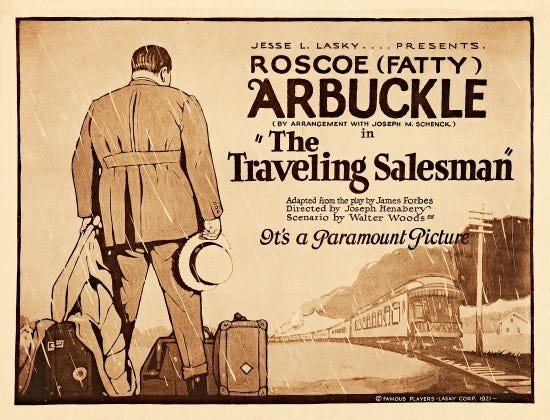# Heuristic Algorithms for the Traveling Salesman Problem# Heuristic Algorithms for the Traveling Salesman Problem

The traveling salesman problem (TSP) involves finding the shortest path that visits n specified locations, starting and ending at the same place and visiting the other n-1 destinations exactly once. To the layman, this problem might seem a relatively simple matter of connecting dots, but that couldn’t be further from the truth.

The TSP is actually one of the most significant problems in the history of applied mathematics. In 1952, three operations researchers (Danzig, Fulkerson, and Johnson, the first group to really crack the problem) successfully solved a TSP instance with 49 US cities to optimality. This breakthrough paved the way for future algorithmic approaches to the TSP, as well as other important developments in the field (like branch-and-bound algorithms). Consequently, it’s fair to say that the TSP has birthed a lot of significant combinatorial optimization research, as well as help us recognize the difficulty of solving discrete problems accurately and precisely.

The TSP’s wide applicability (school bus routes, home service calls) is one contributor to its significance, but the other part is its difficulty. It’s an NP-complete combinatorial problem, and therefore there is no known polynomial-time algorithm that is able to solve all instances of the problem. Some instances of the TSP can be merely understood, as the time required to solve the model optimally might take forever.

Consequently, researchers developed heuristic algorithms to provide solutions that are strong, but not necessarily optimal. In this blog post, I’ll show you the why and the how of two main heuristics for the TSP.

# Understanding the VRP

To help motivate these heuristics, I want to briefly discuss a related problem in operations research, the vehicle routing problem (VRP).

The typical usage of VRP is as follows: given a set of vehicles and a set of locations, and assuming a fixed cost of traversing any location-location pair, find the path that reaches all locations at minimum cost. Although it sounds abstract, it has many applications in the real world (see our blog post on the vehicle routing problem [VRP] for more details).

For instance, in the domain of supply chain, a VRP solution might dictate the delivery strategy for a company that needs to fulfill orders for clients at diverse locations. You could think about it like this: find the cheapest or fastest routes under certain constraints (capacity, time, etc.) for a set of trucks, with each truck starting from a depot, visiting all its clients, and returning to its depot.

This is relevant for the TSP because, in the year 1959, Dantzig and Ramser showed that the VRP is actually a generalization of the TSP — when there are no constraints and only one truck traveling around at a time, the VRP reduces to the TSP. Consequently, a last resort for solving TSPs (when usual TSP heuristic methods aren’t working effectively) is to transform the problem into a VRP.

With that out of the way, let’s proceed to the TSP itself.

# Modeling TSP

Firstly, let’s introduce the TSP model: a directed graph G=(V, A), where V is the set of vertices (locations) to be visited, and cᵢⱼ, (i,j) ∈ A is the cost (usually distance, or a literal dollar cost) of each edge (the path between two locations).

The objective of the TSP is to find the lowest-cost route that satisfies the problem’s four main constraints, specified below.